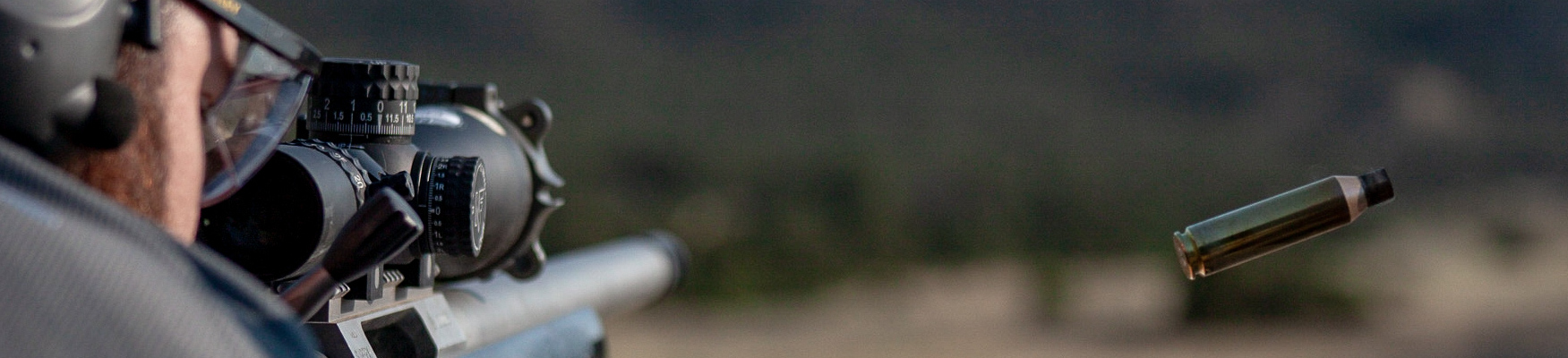# Where Does Wind Matter?

## Where Does Wind Matter?

#### by Dan Periard

Through work on DARPA’s One Shot1 optical wind measurement program, I spent a lot of time looking at how wind, in particular crosswind, affects the flight of a bullet downrange. This article   is intended to investigate how the wind’s influence increases with longer distances, as well as exploring how wind affects the bullet as it moves from the shooter toward the target. I’m actually going to do things in reverse from a typical technical paper, discussing the results first, then walking through the process to get there.

# How much does the wind matter?

First, let’s take a look at how much wind matters at different ranges for a .308 175 grain SMK.   We could use the AB analytics WEZ tool, successively punching in larger ranges to see how the probability of hit changes, but McCoy provides an equation (Equation 1, discussed below) that  can be utilized to give a good first estimate. We can ignore the actual wind speed for now, and  just look at the wind influence (time lag). The units of wind influence are seconds (s), but I find it useful to think of it as meters of deflection per meter per second of wind (m / [m/s]). For example 600m, the wind influence is approximately 0.25 (m/[m/s)); this means that for every increase in  the wind speed of 1 m/s (2.24 MPH), the deflection at 600m increases by 0.25m (9.8 inches)2.

Looking at Figure 1, we see that the influence of the wind increases dramatically with increasing range. For 400m and closer, a 1 m/s wind will deflect the bullet less than 0.1m (4 in); moving to 600m roughly doubles to 0.25m (9.8in). At around 800m, that same wind will blow the bullet 0.47m (18.5 in), or approximately the width of a standard IPSC target. At 1000m, the bullet  moves roughly 0.83m, or over 32 inches. It’s easy to see how much of a factor wind becomes at the further distances.

2 Units of feet can also be used. A 1 ft/s wind would cause a 0.25 ft deflection (3in). As long as units are managed correctly, this approach can take a wind input like 5 MPH and compute the appropriate deflection in whatever units are desired.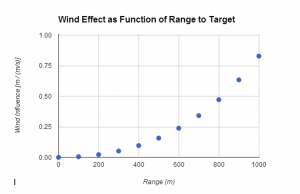Figure 1 ­ The wind’s influence increases dramatically with increasing range.

# Where does wind matter most?

The analysis above assumes an average wind along the entire trajectory, which is great for  basic modeling and estimation, but is rarely representative of real life, with terrain, trees, and other features having a big effect on the wind. McCoy’s Equation 2 (discussed later) provides a tool for looking at how the wind influence values in Figure 1 are actually distributed along the bullet’s path.

Figure 2 shows how the wind influence looks when shooting at 200m, 400m, 600m, 800m, and 1000m. A few items to note: first, the area under these curves, if added up, would equal the values found in Figure 1; second, the early portion of the trajectory has the biggest effect on the round, although as range increases, the middle portion of the range starts to contribute more; lastly, the contribution of the first segment increases with range, which can mostly be attributed  to the increased flight time to the target at the longer ranges.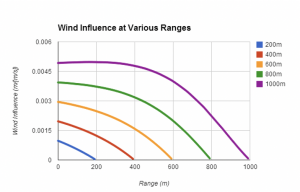Figure 2 ­ A comparison of the wind’s influence at five different ranges

If we break each range into thirds, how much do those segments contribute to the overall effect    of the wind? Figure 3 shows that breakdown: at 200m, the first third accounts for 56% of the influence, the middle contributes 33%, with the third closest to the target roughly 12%. As the distance increases to 1000m, the influence of the first third drops to 44%, the middle third increases to 39%, and the last third grows to 17%. The front portion of the trajectory obviously plays the most significant role in determining the bullet’s deflection, but with increasing range, the middle section needs to be considered as well.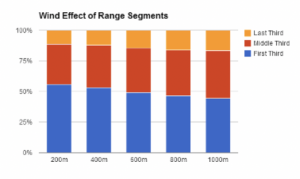Figure 3 ­ The first two­thirds of the trajectory accounts for over 80% of the wind’s influence.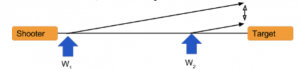Figure 4 ­ The influence of winds early in the path is greater than those closer to target.

I’ve heard a few times that the wind at the target affects the bullet more than the wind at the shooter, because the bullet has slowed down so the wind can push it more. From the analysis above, we can see that this is incorrect, and here’s why: if the bullet is nudged by two identical winds, W1 and W2, as shown in Figure 4, the resulting change in direction from W1 has much more time to act on the bullet, and results in a larger deflection.

In order to achieve the same deflection, W2 would have to be much larger than W1, even accounting for the slowing of the bullet.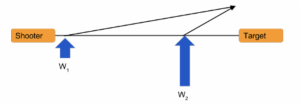Figure 5 ­ It would require a much larger wind downrange to cause the same deflection.

So how did I generate those graphs? Let’s take a look at the equations that govern these effects.

# Background Theory

McCoy’s Modern Exterior Ballistics3 provides a solid discussion on computing how the wind affects a bullet’s flight, with the punch line being that the amount of deflection, D, a bullet experiences from a wind, W, is proportional to the ‘lag time,’ or the difference between the  bullet’s time of flight in a vacuum (the distance to target, R, divided by the muzzle velocity, MV), and the actual time of flight, T.

Equation 1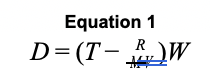This simple formula is further expanded by McCoy to compute how wind in individual sections of the trajectory affects the ultimate hit point of the bullet. The next equation is a little more involved, but you’ll see that it follows the same basic form, and consists of the same basic components.

First, some background: this type of analysis assumes that the bullet’s trajectory has been modeled in discrete steps, as is typical for advanced point mass solvers like Applied Ballistics’. Without going into too much detail, this is how they work:

These solvers take the initial conditions of the bullet, like muzzle velocity, compute the forces on the bullet, and use those forces to determine the bullet’s acceleration. This acceleration results   in a change (decrease) in the bullet’s velocity over a given time period (or step size). During that time period, the bullet has moved some amount, or approximately the velocity multiplied by the time period. The solver then moves to the next time step, using the new velocity to compute the forces, accelerations, and distance traveled. This is repeated until the bullet reaches the target distance.

3 McCoy, Robert L. Modern Exterior Ballistics. 2nd Edition. Schiffer Publishing Ltd. 2012.

Figure 6 illustrates some common notation used to describe the steps above. The letter i is commonly used as an index, and can be thought of as the ith step that the bullet has taken. i­1 is used to describe the step prior to the one you’re interested in, and i+1 for the step following. At each step, there are several parameters that are of interest: distance (Xi), velocity (Vi), and time   of flight (t(Xi)). Solvers generally use i = 0 to describe the starting position (X0 = 0), velocity (V0 = muzzle velocity), and time (t(X0) = 0 ­ no time has passed).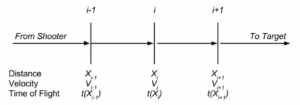Figure 6 ­ Basic Diagram of Steps in Point Mass Solver Output

With this notation in mind, we can use some additional formulae derived by McCoy to compute how a wind speed at step i can change the bullet’s hitpoint all the way at the target. This allows  us to examine how a variable wind affects a bullet, instead of having to use an average wind like in Equation 1. Let’s walk through the formula, then we can discuss its implications further.

Let’s say that frepresents the influence of the wind at the ith position, or how much a unit of    wind in that step changes the bullet’s ultimate hit point. It is related to how much time the bullet spends in that step (the first two terms in equation 2), and a modified version of the time lag from Equation 1. This second part is crucial, as it helps account for how much time a nudge from the bullet at i has to work on the bullet before it gets to the target.

Equation 2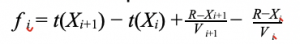Equation 2 isn’t the whole story, however. That simply describes the wind’s influence; it needs to  be multiplied with the wind speed at that location, Wi, to get the deflection at the target. By adding up the individual winds multiplied by the influence values, we can get the total deflection at the target.

Equation 3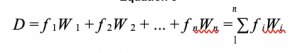# Conclusion

The above analysis examined a few equations that can be used to determine how a bullet will be affected by crosswind as it travels downrange. As expected, the wind’s influence on a bullet increases dramatically as the range to target grows. Perhaps more interesting for many readers   is the description of how the position of wind downrange can influence the flight of a bullet. The first third of the range is the most critical portion to get a good wind call on, although the middle third can’t be discounted, especially as range increases.